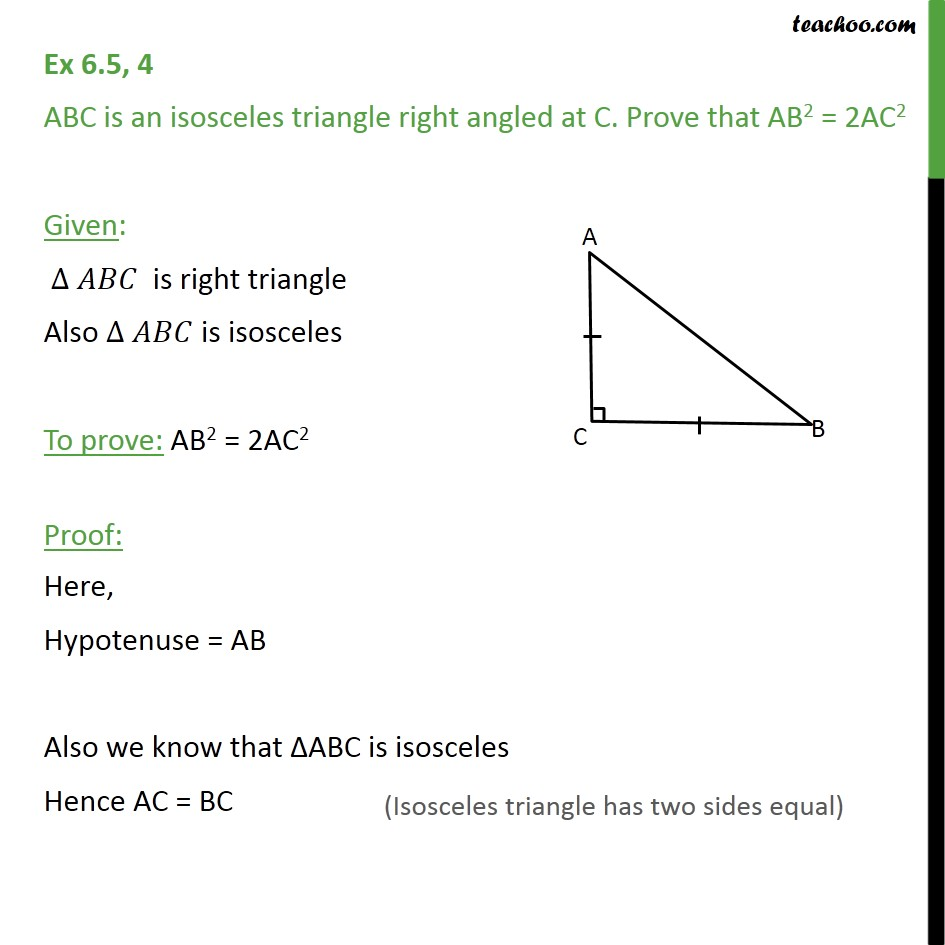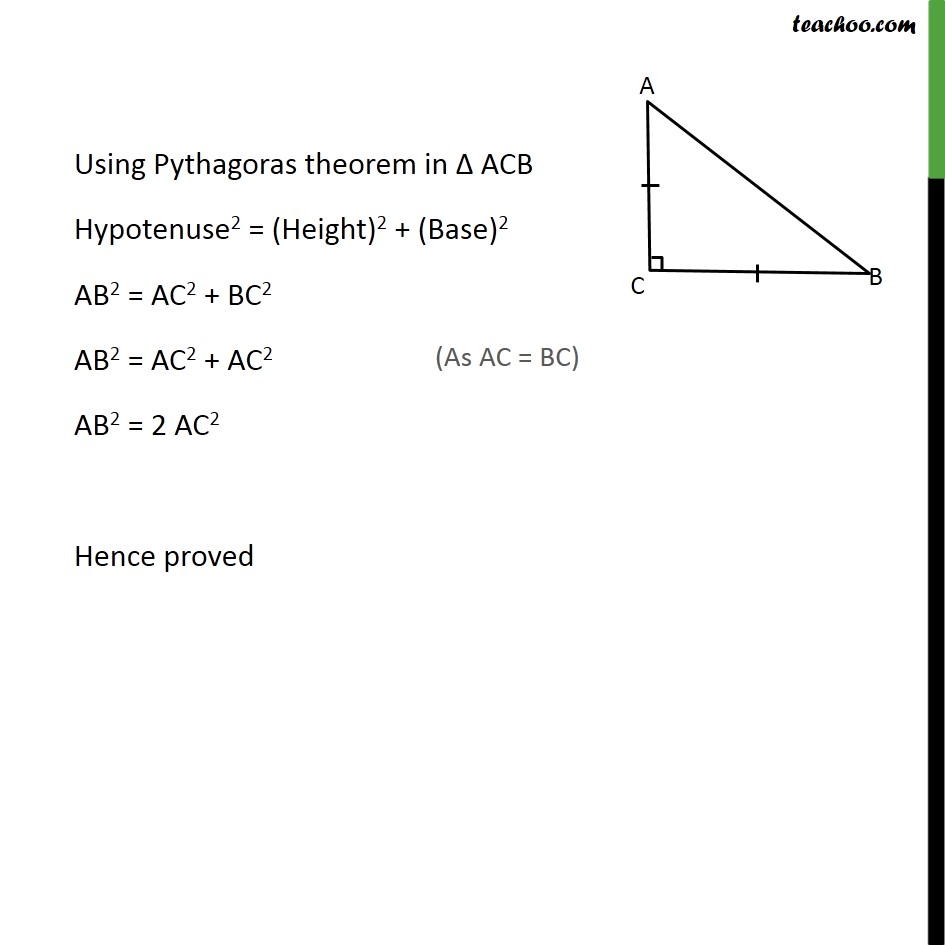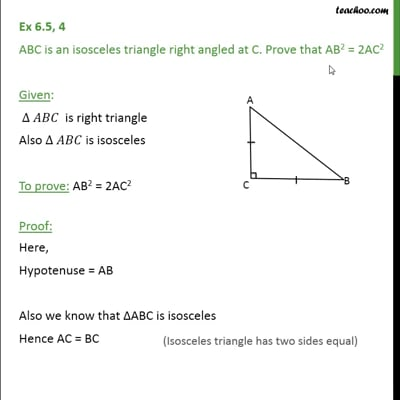Ex 6.5

Chapter 6 Class 10 Triangles (Term 1)
Serial order wiseThis video is only available for Teachoo black users

### Transcript

Ex 6.5, 4 ABC is an isosceles triangle right angled at C. Prove that AB2 = 2AC2 Given: ∆ 𝐴𝐵𝐶 is right triangle Also ∆ 𝐴𝐵𝐶 is isosceles To prove: AB2 = 2AC2 Proof: Here, Hypotenuse = AB Also we know that ΔABC is isosceles Hence AC = BC Using Pythagoras theorem in Δ ACB Hypotenuse2 = (Height)2 + (Base)2 AB2 = AC2 + BC2 AB2 = AC2 + AC2 AB2 = 2 AC2 Hence proved